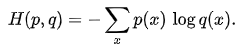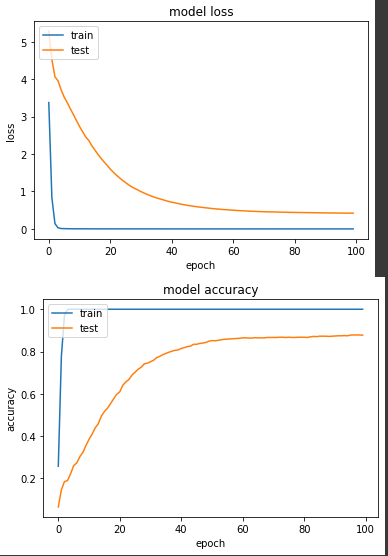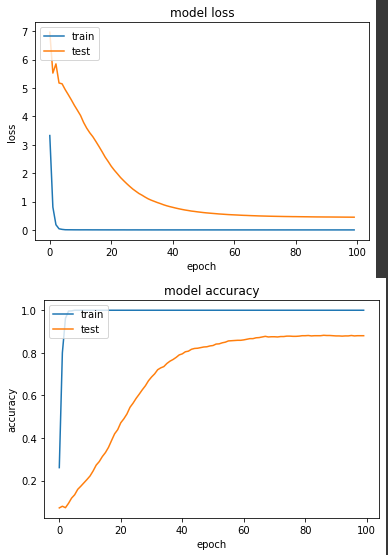#DAY 10
0
AI & Data

## 【10】多分類問題下 Sparse Cross Entropy 與 Categorical Cross Entropy 的用法差異

Colab連結``````[0 0 0 0 0 0 1 0 0 0]
[0 0 0 0 0 0 0 1 0 0]
[1 0 0 0 0 0 0 0 0 0]
[0 0 0 0 1 0 0 0 0 0]
[0 0 0 0 0 0 0 0 1 0]
[0 1 0 0 0 0 0 0 0 0]
``````

``````[1 0 0 1 0 0 1]  # 一位有鬍子表情生氣的男性
[0 1 1 0 0 1 0]  # 一位有眼鏡表情高興的女性
[0 1 0 0 1 0 0]  # 一位表情難過的女性
[1 0 0 1 0 1 1]  # 一位有鬍子有眼鏡表情生氣的男性
``````

``````def normalize_img(image, label):
image = tf.cast(image, tf.float32)
image = tf.image.resize(image, (224,224))
return image / 255., label
``````
``````base = tf.keras.applications.MobileNetV2(input_shape=(224, 224, 3), include_top=False, weights='imagenet')
net = tf.keras.layers.GlobalAveragePooling2D()(base.output)
net = tf.keras.layers.Dense(NUM_OF_CLASS)(net)

model = tf.keras.Model(inputs=[base.input], outputs=[net])

model.compile(
optimizer=tf.keras.optimizers.SGD(LR),
loss=tf.keras.losses.SparseCategoricalCrossentropy(from_logits=True),
metrics=[tf.keras.metrics.SparseCategoricalAccuracy()],
)

start = timeit.default_timer()
history = model.fit(
ds_train,
epochs=EPOCHS,
validation_data=ds_test,
verbose=True)
``````

``````loss: 5.0338e-04 - sparse_categorical_accuracy: 1.0000 - val_loss: 0.4206 - val_sparse_categorical_accuracy: 0.8775
````````````def normalize_img_one_hot(image, label):
image = tf.cast(image, tf.float32)
image = tf.image.resize(image, (224,224))
return image / 255., tf.one_hot(label, NUM_OF_CLASS)
``````
``````def normalize_img_one_hot(image, label):
image = tf.cast(image, tf.float32)
image = tf.image.resize(image, (224,224))
return image / 255., tf.one_hot(label, NUM_OF_CLASS)

ds_train = train_split.map(
normalize_img_one_hot, num_parallel_calls=tf.data.experimental.AUTOTUNE)
ds_train = ds_train.cache()
ds_train = ds_train.shuffle(SHUFFLE_SIZE)
ds_train = ds_train.batch(BATCH_SIZE)
ds_train = ds_train.prefetch(tf.data.experimental.AUTOTUNE)

ds_test = test_split.map(
normalize_img_one_hot, num_parallel_calls=tf.data.experimental.AUTOTUNE)
ds_test = ds_test.batch(BATCH_SIZE)
ds_test = ds_test.cache()
ds_test = ds_test.prefetch(tf.data.experimental.AUTOTUNE)
``````

``````loss: 3.9605e-04 - categorical_accuracy: 1.0000 - val_loss: 0.4483 - val_categorical_accuracy: 0.8804
``````